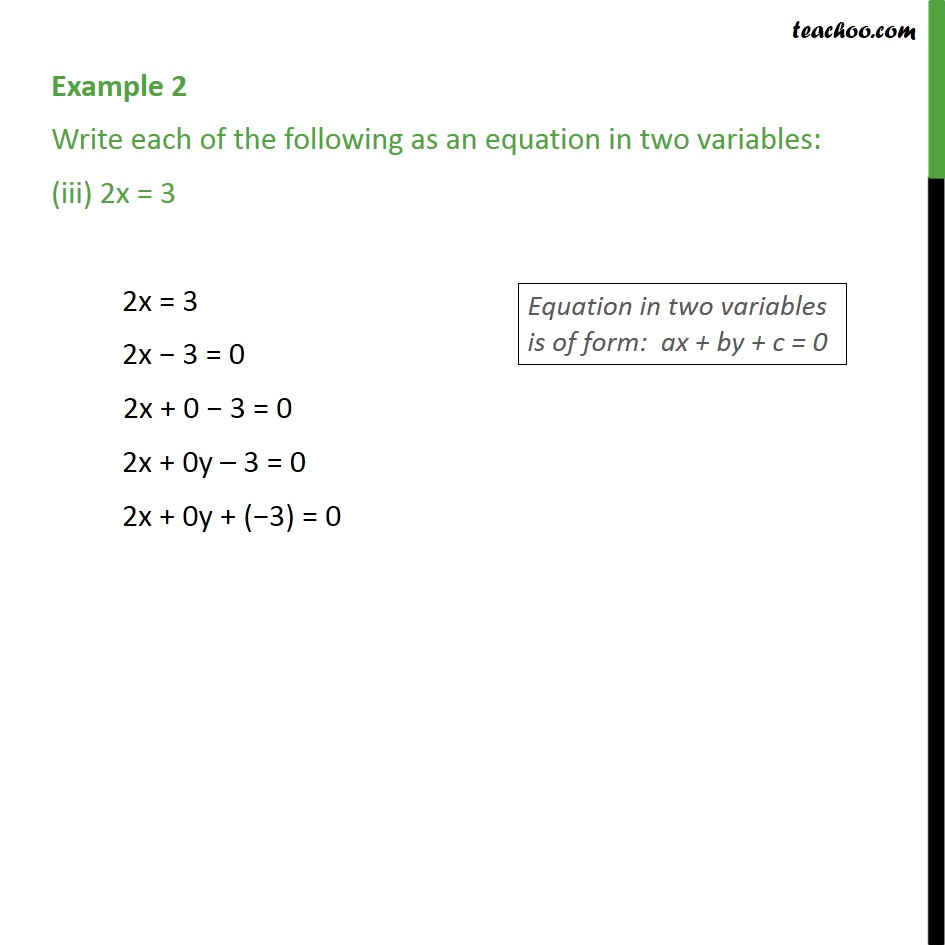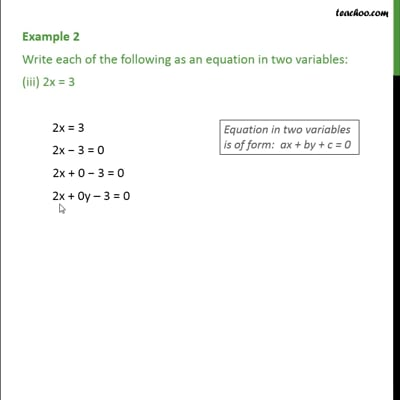Examples

Chapter 4 Class 9 Linear Equations in Two Variables
Serial order wiseThis video is only available for Teachoo black users

Introducing your new favourite teacher - Teachoo Black, at only ₹83 per month

### Transcript

Example 2 Write each of the following as an equation in two variables: (iii) 2x = 3 2x = 3 2x 3 = 0 2x + 0 3 = 0 2x + 0y 3 = 0 2x + 0y + ( 3) = 0